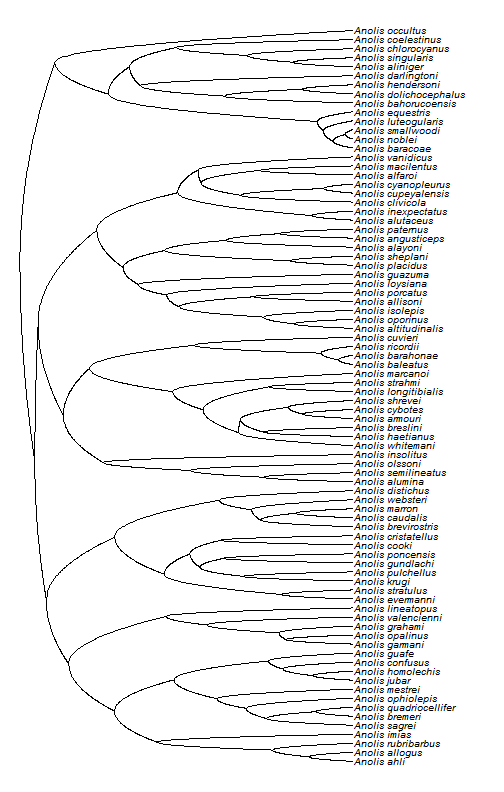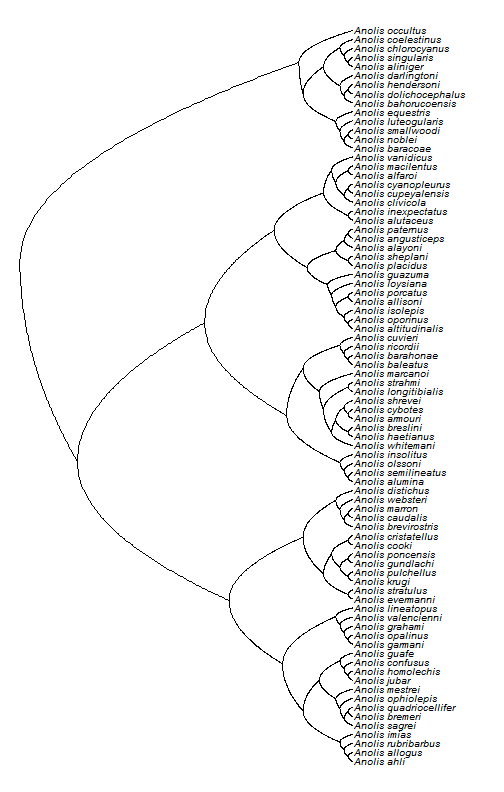## Monday, August 10, 2015

### Neglected phytools functions I: roundPhylogram

I'm not sure that this is destined to become a series, but in performing my latest “major”/minor update to phytools (and seeing as so doing meant modifying almost every source file in the phytools package), I stumbled across more than a few phytools functions that I'm not sure I've ever seen used outside the pages of this blog.

Here's one that I particularly like: `roundPhylogram`.

This function plots a tree with rounded (parabolic, more precisely) edges. Here's what I mean by that:

``````library(phytools)
data(anoletree)
roundPhylogram(anoletree,ftype="i",lwd=1,fsize=0.8)
``````As is commonly true, we get a nicer looking figure - that is, one without aliasing - if we export directly to a PDF:

``````pdf(file="roundPhylogram-Anolis.pdf",width=7,height=11)
roundPhylogram(anoletree,ftype="i",lwd=1,fsize=0.7)
dev.off()
``````
``````## windows
##       2
``````

which can be seen here.

If we want to ignore branch lengths, as is commonly done for this type of tree plot - well, we might call that a round

``````roundCladogram<-function(x,...){
x\$edge.length<-NULL
roundPhylogram(x,...)
}1.